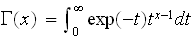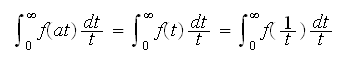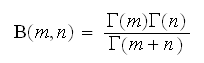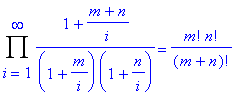## Why is Gamma(n) = (n-1)! and not Gamma(n) = n! ?

A possible answer was given by Robin Chapman in the newsgroup sci.math, in a response to a remark of David W. Cantrell, quoting from C. Lanczos' opening paragraph in "A precision approximation of the gamma function":

"... Gamma(n+1) = n! The normalization of the gamma function to Gamma(n+1) instead of Gamma(n) is due to Legendre and void of any rationality. This unfortunate circumstance compels us to utilize the notation z! instead of Gamma(z+1)."

The Gamma function is the Mellin transform of the exponential function.The Mellin transform of f beingWell you might ask, why not absorb the final 1/t into the t^s and change s-1 to s? The point though is that dt/t should be inseparable in this context as dt/t is the Haar measure on the multiplicative group of the positive reals. That is.
This becomes important when studying the zeta function and its functional equation. One gets nice integral representations of Gamma(s)zeta(s) and Gamma(s/2)zeta(s), but not of s! zeta(s) and (s/2)! zeta(s).

See: sci.math.

But note also that for Re(s) > 1 the Mellin transform can be written asand for 0 < Re(s) < 1 the Mellin transform isHere {x} is the fractional part of x. Thus the Mellin transform does not necessarily suggest the use of the Gamma function over the factorial function.

In any case, the Mellin transform does not explain the reason why Euler changed his definition between 1729/30 and 1768. Perhaps it was the relation to the Beta function (also introduced by Euler) which Euler wanted to express by the identityHowever, again this is not absolutely convincing because of the following formula which might be known to Euler and is also a very useful representation, although the right hand side does not bear a special nameStill, there is another way to look at the relation between the factorial function and the Gamma function when we look at the (generalized) factorial powers -- z and w arbitrary complex numbers:In this setup, which can be found in Graham, Knuth, Patashnik 'Concrete Mathematics', (2. ed., p. 211), things look more like a duality. GKP remark: "... the Gamma function, which relates to ordinary factorials somewhat as rising powers relate to falling powers."

```::::::::::::::::::::::::::::::::::::::::::::::::
::: From sci.math "Gamma function question"  :::
::: 22 Jun. 2007                             :::
::::::::::::::::::::::::::::::::::::::::::::::::
::::::::::::::::::::::::
::: David C. Ullrich :::
::::::::::::::::::::::::

A possible reason is this: dt/t is the Haar measure on the
group of positive reals with multiplication for the group
operation. If you don't know what that means, what it amounts
to is that integrals of the form

int_0^infinity f(t) dt/t

transform very nicely under various changes of variables,
for example (writing int for int_0^infinity) if c > 0 and
a is real, a <> 0 then

int f(t) dt/t = int f(ct) dt/t = int f(1/t) dt/t
= |a| int f(t^a) dt/t
= int_{-infinity}^infinity f(e^t) dt,

etc. With dt instead of dt/t all those formulas look more
complicated. I'm not saying that that's _the_ reason, but
I think of the definition as

Gamma(x) = int e^{-t} t^x dt/t

and it makes more sense, at least to me.

(Even if those transformations don't come up, thinking about
int f(t) dt/t instead of int f(t) dt just seems "natural"
from the right point of view.)

If you know a little real analysis, in particular what an
"L^p space" is, then read on:

Maybe the reason it makes sense to me to think of it this way
is because of various formulas that come up in harmonic analysis.
For example, in one characterization of "Besov spaces"
you see a definition of the form

(*)  something = [int (f(t)^p)/t^(ap+1) dt]^{1/p],

which is supposed to interpreted as

(**) sup_t f(t)/t^a

when p = infinity. When I look at the formula written like that
it makes no sense to me, it's not clear why (*) should become (**)
for p = infinity, and I can't imagine how anyone would keep it
straight. Instead I write the formula as

(*') [int (f(t)/t^a)^p dt/t]^(1/p)

and it makes perfect sense - it's just an L^p norm with respect
to the measure dt/t (this makes the formula much easier for me
to remember and it also makes it clear why it becomes (**)
for p = infinity.)

::::::::::::::::::::::::::
::: Zdislav V. Kovarik :::
::::::::::::::::::::::::::

The chapter (of Functional Equations) considers summation of
a function f(x) as a (right) inverse of the forward difference,
not of the backward difference.

In formulas, F(x) is a sum of f(x) if

F(x+1)-F(x) = f(x)

(in an appropriate domain).

There are many such F's to a given f, so extra conditions may
be studied. In this terminology, a sum of ln(x) is ln(Gamma(x)).
(Same as Gamma(x+1)-x*Gamma(x).) Very neat, I suggest.

Remark: Given an extra condition that a sum of ln(x) be convex
("concave up") for x>0, and have value 0 at x=1, we end up with
the one and only ln(Gamma(x)). That is Bohr-Mollerup Theorem.

Exercise: Why convexity? (The question persists for Gamma(x+1),
too). This is not a frivolous condition, just an extension of
the discrete logarithmic convexity of the factorial.

The exercise is: Using operations on integers alone (no Calculus),
show that whenever a, b, c are integers, 1<=a<=b<=c, we have
(c!/b!)^a * (a!/c!)^b * (b!/a!)^c <=1. (When does equality occur?
And how does it relate to log-convexity?)
```

On MathOverflow this question was discussed on 10 Apr 2010.
Why is the gamma function shifted from the factorial by 1?Back to the Homepage of Factorial Algorithms.